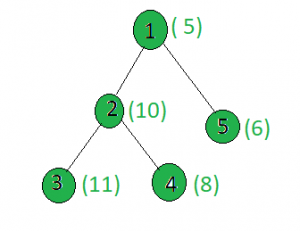Skip to content
Related Articles
Find the node whose xor with x gives minimum value
• Difficulty Level : Medium
• Last Updated : 16 Apr, 2021

Given a tree, and the weights of all the nodes and an integer x, the task is to find a node i such that weight[i] xor x is minimum.
Examples:

Input:x = 15
Output:
Node 1: 5 xor 15 = 10
Node 2: 10 xor 15 = 5
Node 3: 11 xor 15 = 4
Node 4: 8 xor 15 = 7
Node 5: 6 xor 15 = 9

Approach: Perform dfs on the tree and keep track of the node whose weighted xor with x gives the minimum value.
Below is the implementation of above approach:

## C++

 `// C++ implementation of the approach``#include ``using` `namespace` `std;` `int` `minimum = INT_MAX, x, ans;` `vector<``int``> graph;``vector<``int``> weight(100);` `// Function to perform dfs to find``// the minimum xored value``void` `dfs(``int` `node, ``int` `parent)``{``    ``// If current value is less than``    ``// the current minimum``    ``if` `(minimum > (weight[node] ^ x)) {``        ``minimum = weight[node] ^ x;``        ``ans = node;``    ``}``    ``for` `(``int` `to : graph[node]) {``        ``if` `(to == parent)``            ``continue``;``        ``dfs(to, node);``    ``}``}` `// Driver code``int` `main()``{``    ``x = 15;` `    ``// Weights of the node``    ``weight = 5;``    ``weight = 10;``    ``weight = 11;``    ``weight = 8;``    ``weight = 6;` `    ``// Edges of the tree``    ``graph.push_back(2);``    ``graph.push_back(3);``    ``graph.push_back(4);``    ``graph.push_back(5);` `    ``dfs(1, 1);` `    ``cout << ans;` `    ``return` `0;``}`

## Java

 `// Java implementation of the approach``import` `java.util.*;``import` `java.lang.*;` `class` `GFG``{` `    ``static` `int` `minimum = Integer.MAX_VALUE, x, ans;` `    ``@SuppressWarnings``(``"unchecked"``)``    ``static` `Vector[] graph = ``new` `Vector[``100``];``    ``static` `int``[] weight = ``new` `int``[``100``];` `    ``// This block is executed even before main() function``    ``// This is necessary otherwise this program will``    ``// throw "NullPointerException"``    ``static``    ``{``        ``for` `(``int` `i = ``0``; i < ``100``; i++)``            ``graph[i] = ``new` `Vector<>();``    ``}` `    ``// Function to perform dfs to find``    ``// the minimum xored value``    ``static` `void` `dfs(``int` `node, ``int` `parent)``    ``{` `        ``// If current value is less than``        ``// the current minimum``        ``if` `(minimum > (weight[node] ^ x))``        ``{``            ``minimum = weight[node] ^ x;``            ``ans = node;``        ``}``        ``for` `(``int` `to : graph[node])``        ``{``            ``if` `(to == parent)``                ``continue``;``            ``dfs(to, node);``        ``}``    ``}` `    ``// Driver Code``    ``public` `static` `void` `main(String[] args)``    ``{``        ``x = ``15``;` `        ``// Weights of the node``        ``weight[``1``] = ``5``;``        ``weight[``2``] = ``10``;``        ``weight[``3``] = ``11``;``        ``weight[``4``] = ``8``;``        ``weight[``5``] = ``6``;` `        ``// Edges of the tree``        ``graph[``1``].add(``2``);``        ``graph[``2``].add(``3``);``        ``graph[``2``].add(``4``);``        ``graph[``1``].add(``5``);` `        ``dfs(``1``, ``1``);` `        ``System.out.println(ans);``    ``}``}` `// This code is contributed by SHUBHAMSINGH10`

## C#

 `// C# implementation of the approach``using` `System;``using` `System.Collections.Generic;` `class` `GFG``{` `static` `int` `minimum = ``int``.MaxValue, x, ans;` `static` `List> graph = ``new` `List>();``static` `List<``int``> weight = ``new` `List<``int``>();`  `// Function to perform dfs to find``// the minimum value``static` `void` `dfs(``int` `node, ``int` `parent)``{``    ``// If current value is less than``    ``// the current minimum``    ``if` `(minimum > (weight[node] ^ x))``    ``{``        ``minimum = weight[node] ^ x;``        ``ans = node;``    ``}``        ` `    ``for` `(``int` `i = 0; i < graph[node].Count; i++)``    ``{``        ``if` `(graph[node][i] == parent)``            ``continue``;``        ``dfs(graph[node][i], node);``    ``}``}` `// Driver code``public` `static` `void` `Main()``{``    ``x = 15;` `    ``// Weights of the node``    ``weight.Add(0);``    ``weight.Add(5);``    ``weight.Add(10);``    ``weight.Add(11);;``    ``weight.Add(8);``    ``weight.Add(6);``    ` `    ``for``(``int` `i = 0; i < 100; i++)``    ``graph.Add(``new` `List<``int``>());` `    ``// Edges of the tree``    ``graph.Add(2);``    ``graph.Add(3);``    ``graph.Add(4);``    ``graph.Add(5);` `    ``dfs(1, 1);``    ``Console.Write( ans);``}``}` `// This code is contributed by SHUBHAMSINGH10`

## Python3

 `# Python implementation of the approach``from` `sys ``import` `maxsize` `minimum, x, ans ``=` `maxsize, ``None``, ``None` `graph ``=` `[[] ``for` `i ``in` `range``(``100``)]``weight ``=` `[``0``] ``*` `100` `# Function to perform dfs to find``# the minimum xored value``def` `dfs(node, parent):``    ``global` `x, ans, graph, weight, minimum` `    ``# If current value is less than``    ``# the current minimum``    ``if` `minimum > weight[node] ^ x:``        ``minimum ``=` `weight[node] ^ x``        ``ans ``=` `node` `    ``for` `to ``in` `graph[node]:``        ``if` `to ``=``=` `parent:``            ``continue``        ``dfs(to, node)` `# Driver Code``if` `__name__ ``=``=` `"__main__"``:` `    ``x ``=` `15` `    ``# Weights of the node``    ``weight[``1``] ``=` `5``    ``weight[``2``] ``=` `10``    ``weight[``3``] ``=` `11``    ``weight[``4``] ``=` `8``    ``weight[``5``] ``=` `6` `    ``# Edges of the tree``    ``graph[``1``].append(``2``)``    ``graph[``2``].append(``3``)``    ``graph[``2``].append(``4``)``    ``graph[``1``].append(``5``)` `    ``dfs(``1``, ``1``)` `    ``print``(ans)` `# This code is contributed by``# sanjeev2552`

## Javascript

 ``
Output:
`3`

Attention reader! Don’t stop learning now. Get hold of all the important DSA concepts with the DSA Self Paced Course at a student-friendly price and become industry ready.  To complete your preparation from learning a language to DS Algo and many more,  please refer Complete Interview Preparation Course.

In case you wish to attend live classes with industry experts, please refer Geeks Classes Live

My Personal Notes arrow_drop_up